# Game Theory (Normal-form Game) | Set 7 (Graphical Method [M X 2] Game)

The payoff matrix of an M * 2 game consists of M rows and two columns. This article will discuss how to solve an M * 2 game by graphical method. Also, this article will discuss if more than two lines intersect the same point in the graph then how can a 2 * 2 payoff matrix be formed.
Consider the below problem: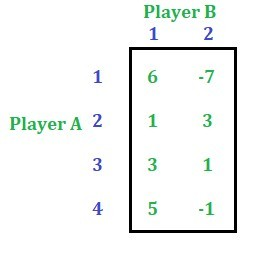Solution: First check whether the problem has got saddle point or not. This game has no saddle point.

Step 1: Reduce the size of the payoff matrix of player A by applying the dominance property, if it exists. The size is being reduced just to simplify the problem. The game can be solved without reducing the size also.
In this problem the dominance property is not applicable. We cannot simplify this matrix more than this. So, we are remain with the following game.Step 2: Let y be the probability of selection of alternative 1 by player B and (1 – y) be the probability of selection of alternative 2 by player B.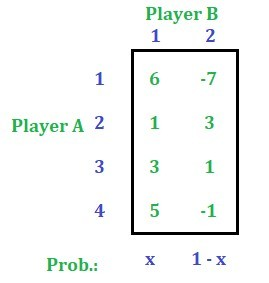Derive the expected gain function of player B with respect to each of the alternatives of player A. See the table below.
Along with find the value of the gain when y = 0 and y = 1. See the table below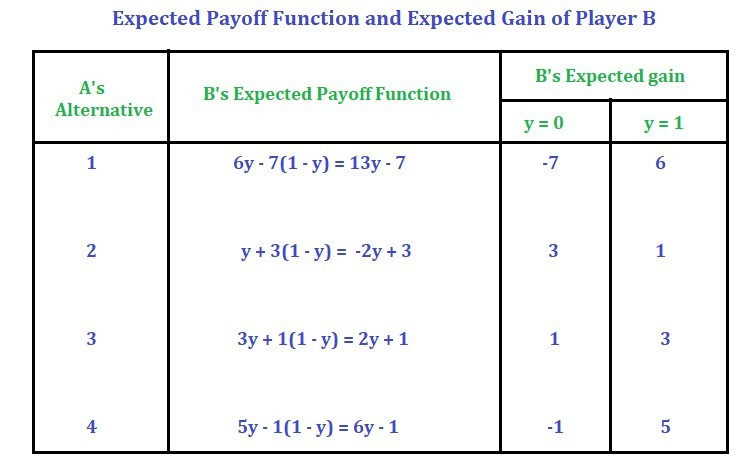Step 3: Plot the gain function on a graph by assuming a suitable scale. Keep y on X-axis and the gain on Y-axis.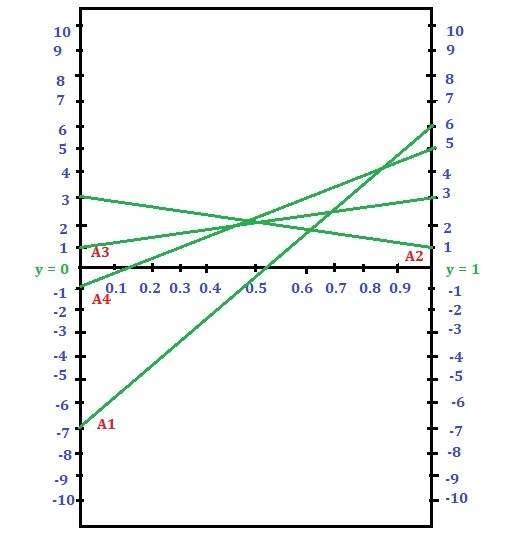Step 3: Find the lowest intersection point in the upper boundary of the graph –> Minimax point.
ABCD is the upper boundary in the given graph. There are four intersection points where B is the lowest intersection. So this point is called Minimax point.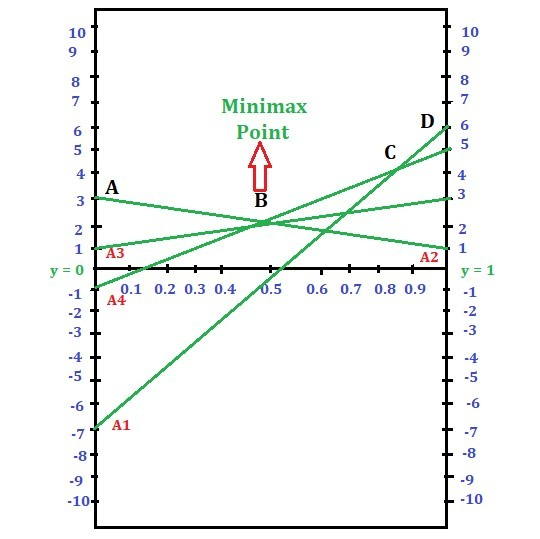Since there are more than two lines passing through the lowest intersection point, we will identify any two lines with opposite slopes passing through that point. Then form a 2 * 2 payoff matrix.
A4, A3 and A2 are three lines passing through the point B. So, among these three lines A2 and A3 are of opposite slopes, and A2 and A4 are of opposite slopes. We can select any of the two pairs of lines. Here we select A2 and A4. Then the payoff matrix will be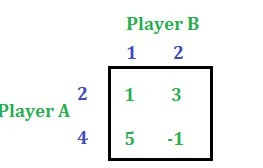After solving the game we get,
Probabilities of strategies of Player A = [0, 3/4, 0, 1/4]
Probabilities of strategies of player B = [1/2, 1/2]
And the value of the game is 2

Attention reader! Don’t stop learning now. Get hold of all the important DSA concepts with the DSA Self Paced Course at a student-friendly price and become industry ready.

My Personal Notes arrow_drop_upPossess good Mathematical and Statistical Foundation Data Science Enthusiast Addicted to Python

If you like GeeksforGeeks and would like to contribute, you can also write an article using contribute.geeksforgeeks.org or mail your article to contribute@geeksforgeeks.org. See your article appearing on the GeeksforGeeks main page and help other Geeks.

Please Improve this article if you find anything incorrect by clicking on the "Improve Article" button below.